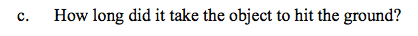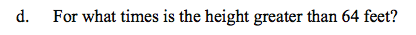Home > CCA2 > Chapter Ch10 > Lesson 10.2.2 > Problem10-130

10-130.
1. For an object shot into the air, its height h in feet above the ground after t seconds is given by the equation h = 80t – 16t2. Use this equation to answer the following questions. Homework Help ✎

1. At what times is the object on the ground?

2. For what domain is this function reasonable?

3. How long did it take the object to hit the ground?

4. For what times is the height greater than 64 feet?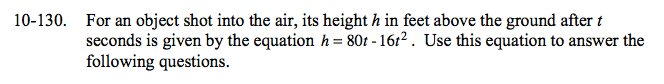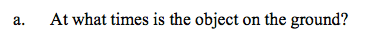This is when the height is zero, or h = 0.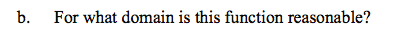This is an object shot into the air, hence its height is always positive or 0. For what times is h ≥ 0?